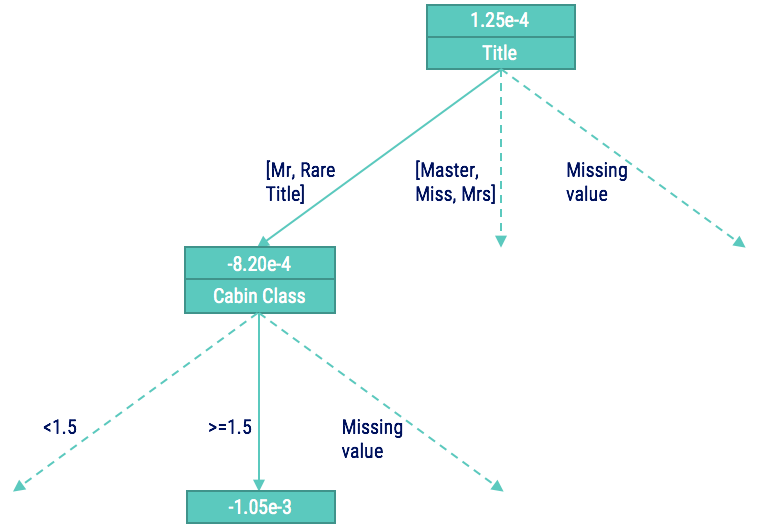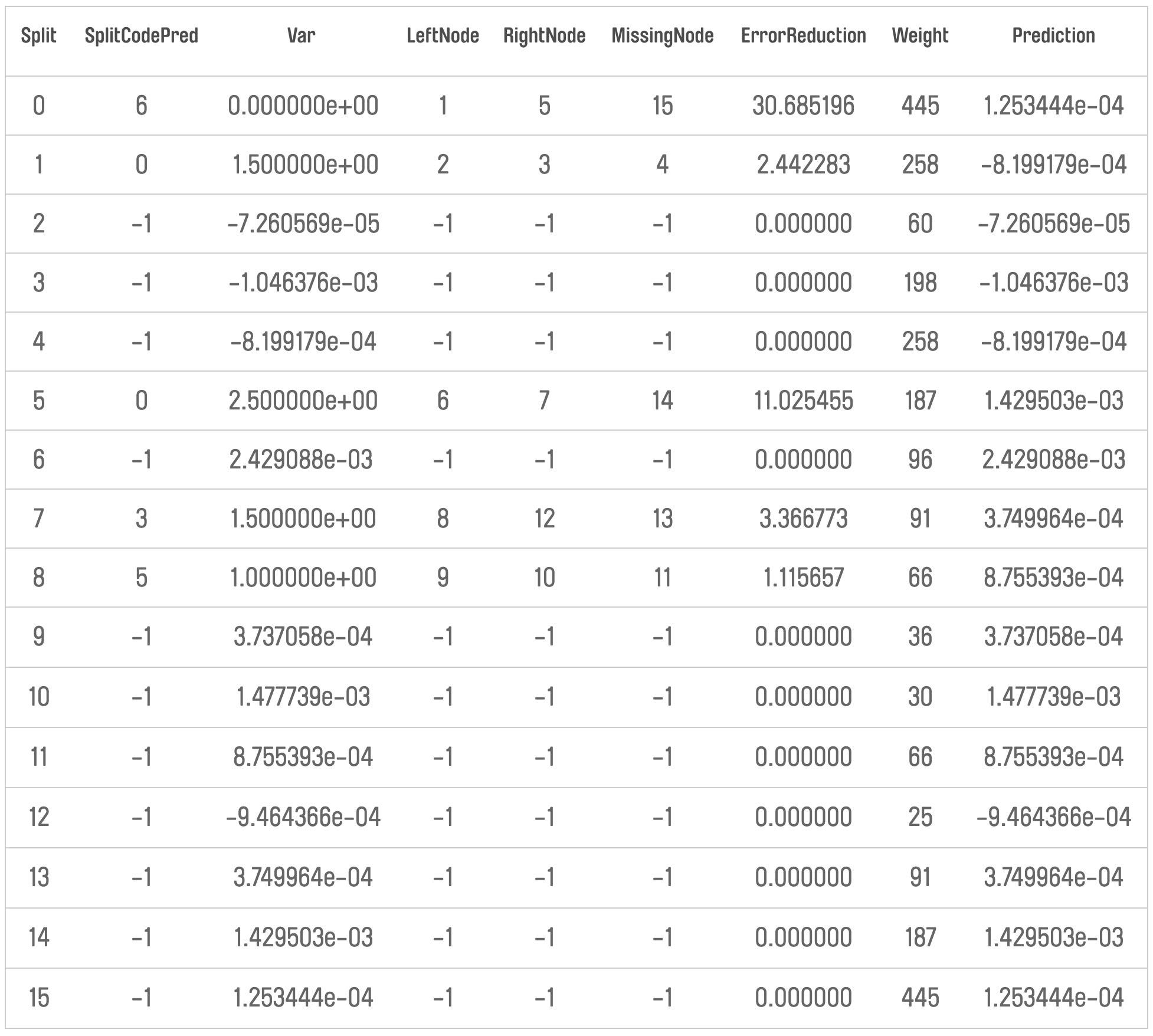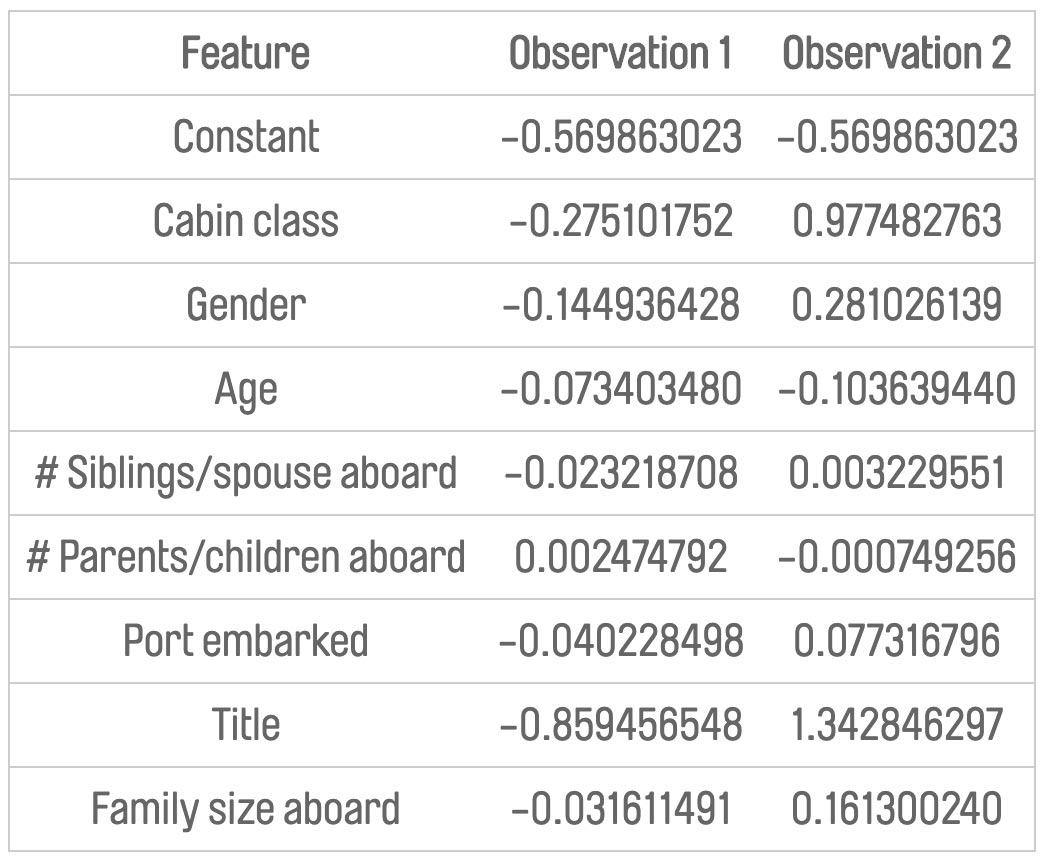### Gradient boosting can be seen as a “black box”

Gradient boosting is a great technique for fitting predictive models and one that data scientists frequently use to get that extra bit of performance above traditional regression fitting. The downside of gradient boosting is that it is less interpretable – it is sometimes viewed as a “black box” model. This gives rise to two problems:

• Understanding the overall model structure
• Understanding individual model predictions

Let’s take a look at the second problem in a bit more detail. For a given observation, we want to be able to explain why the model has reached its prediction.

This is an important consideration for the team at MarketInvoice. Having an understanding behind the model prediction benefits both the business user and the data scientist. For the business user, it provides insight into what characteristics are most important and builds buy-in of the model decision. For the data scientist, it’s useful in the investigation of incorrect prediction cases as a means of identifying if there are any underlying issues with the features in the model.

### We want to understand what features have contributed to a prediction

As an example, let’s suppose that we have developed a gradient boosted model using the gbm function in R on the Titanic dataset from Kaggle to predict whether a passenger survives. This is a classification problem (survival or no survival), so we have used the Bernoulli distribution as the loss function and the resulting model is an ensemble of decision trees. The prediction for an observation is calculated by summing a constant and all the values at the terminal node of each tree, then transforming back from the log-odds space.

Let’s consider the predictions of our model for two observations:These observations have predicted survival probabilities that are at opposite ends of the spectrum so it’s natural to question why. We want to understand how the different features are impacting the prediction – and to what extent.

The internal structure of the trees explains the contribution of each variable In thinking about the contribution of each feature to a prediction, it’s useful to first take a step back and remind ourselves how the model calculates a predicted value. The predicted value is calculated by summing a constant and all the values at the terminal nodes of the trees, then applying the inverse logit transform. The value at the terminal node of each tree is determined by walking through the tree using the observed values of each feature.

To better visualise this, we use the pretty.gbm.tree function. See below for the code and output of the first tree in the ensemble:Using this output and the c.splits object (which contains information on the splits for categorical variables) we can walk through the tree for our observation 1. The diagram below shows the portion of the tree that we walk through:Notice that at each node in the tree we have the predicted value up to that point in the tree. Therefore, we can calculate the impact that each split had by looking at the predicted value before and after the split. We can do this up to the terminal node of each tree, at which point the value is actually used as part of the sum of values for the probability calculation.

For example, in the tree above for observation 1, the Title feature had the contribution of changing the predicted value from 1.25e-4 to -8.20e-4. In order to understand the overall contribution of a feature, we need to sum these calculations across all of the trees in the ensemble.

When we do this we get the below feature contribution values for our observations:The tree values are in the log-odds space, so if we sum these contributions up and transform back to the response space we get the probabilities of 12% and 90% respectively. A positive contribution increases the probability of survival, whereas a negative contribution reduces the probability.

For the above observations, we can conclude the following:

• Observation 1: The Title being “Mr” is the feature which is reducing the probability of survival the most, followed by the Cabin Class being 3. All features apart from # parents/children are reducing the predicted probability for this observation.
• Observation 2: Most features are increasing the probability of survival, with the Title of “Mrs” and the Cabin Class 1 doing this to the greatest extent.

### Our calculation makes gradient boosted models more understandable

In this example, we’ve seen how the internal structure of a gradient boosted model can help us to summarise the contribution of each feature to the overall prediction. Using these ideas, we can build tools which give us powerful insights into the reasoning behind models that are sometimes shrouded in mystery.